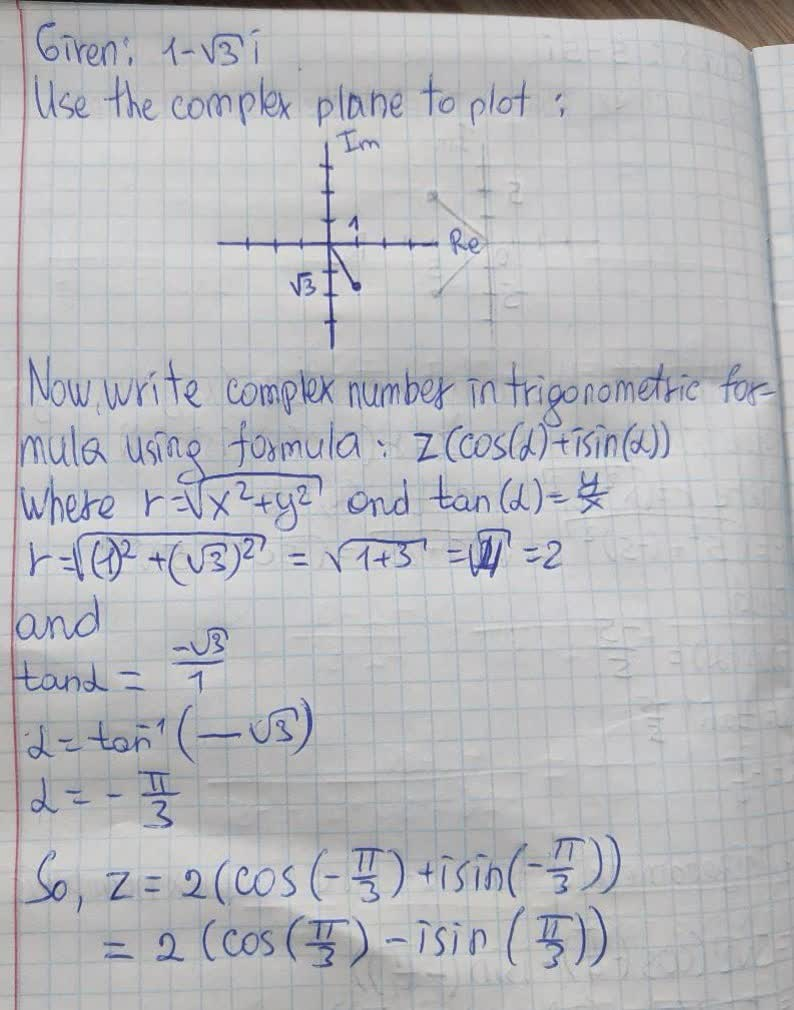Question# Plot each complex number in the complex plane: a. z =

Complex numbers
ANSWEREDPlot each complex number in the complex plane:
a. z = 2 + 3i
b. z = -3 - 5i
c. z = -4
d. z = -i.